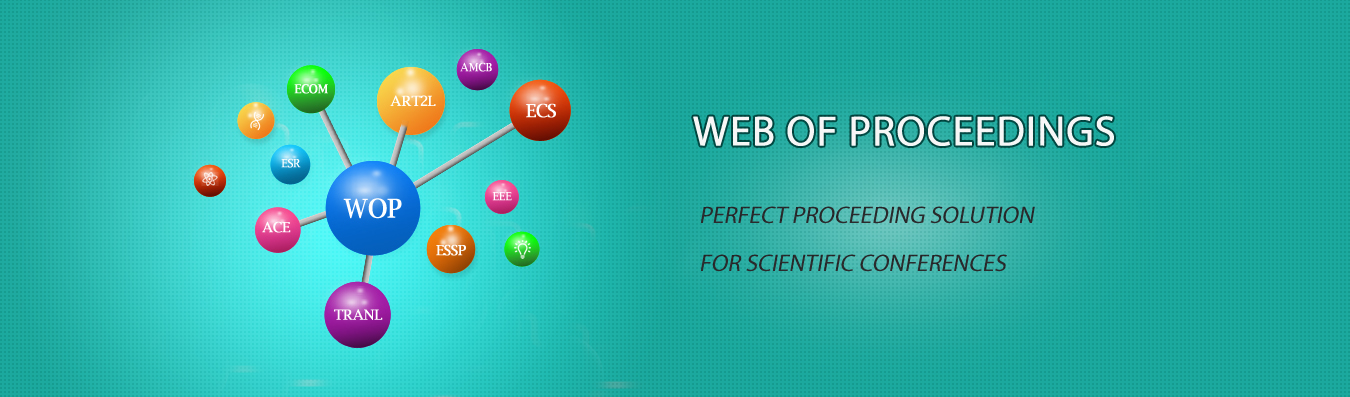The best way to conference proceedings by Francis Academic Press### Linearization Model and State Space Model Analysis of Inverted Pendulum System

DOI: 10.25236/iwmecs.2020.076

#### Author(s)

Xiao Cong, Liming Gong, Junwei Lei, and Yuliang Chen

Xiao Cong

#### Abstract

In this paper, aiming at the nonlinear inverted pendulum system model, the linearization model is analyzed, and the small perturbation method is mainly used to linearize the inverted pendulum system model. On this basis, the state space model is analyzed, and the state space equation is established by choosing appropriate physical variables as state variables. Finally, the matrix state space equation description form is obtained, which provides a good model basis for the subsequent digital simulation analysis and step response analysis of the system

#### Keywords

Pendulum System, Simulation, Linearization, State Space, Model